# Mathematics - Signals And Systems - Laplace Transform Properties

in StemSocial5 months ago[Image1]

## Introduction

Hey it's a me again @drifter1!

Today we continue with my mathematics series about Signals and Systems in order to cover the Laplace Transform Properties.

So, without further ado, let's get straight into it!

## Laplace Transform

In the previous article we covered how we end up with the Laplace Transform, and why it can be used to analyze systems that don't converge using the Fourier Transform. The Laplace Transform is basically a generalization of the Fourier Transform, where the exponent is a complex number. We also mentioned that the Laplace Transform can sometimes simplify the calculations for LTI Systems, and more specifically higher-order systems.

By definition, the Laplace Transform is calculated using: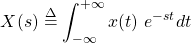The inverse Laplace Transform is defined as follows: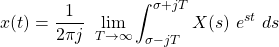Of course the Laplace Transform has similar properties to the Fourier Transform, which of course include linearity, time- and frequency-shifting, time-scaling, conjugation, differentiation etc. And it's also interesting and neseccary to cover how the ROC is affected when such properties come into play! So, let's get into them...

## Laplace Transform Properties

### Linearity

Consider the following two Laplace Transforms: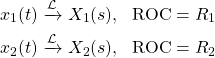For any linear combination: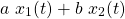the resulting Laplace Transform is given by: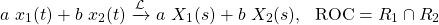where the ROC is the intersection of the two individual ROCs.

### Time- and Frequency-Shifting

Let's consider the Laplace Transform: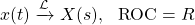The Laplace Transform of the time-shifted x(t - t0) has the same ROC and is given by: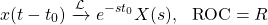whilst the frequency-shifted es0t x(t) has a different ROC and is given by: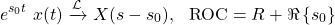### Time-Scaling

Time-scaling a signal by a> yields the following Laplace Transform: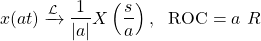As such time-inversion (multiply by -1) yields an inversion of the ROC: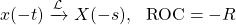### Complex Conjugation

The complex-conjugate x*(t) has the same ROC as the original signal, and its Laplace Transform is defined as: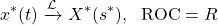When the signal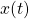is real, the result is basically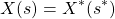.

### Multiplication and Convolution

Similar to the Fourier Transform, Multiplication turns into Convolution, whilst Convolution turns into Multiplication. As such this pair of properties is defined as: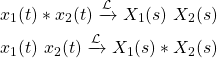with the ROC at least including the union of the two individual ROCs: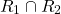The actual formula for calculating the convolution is a little bit different than what we are used to, but let's not get into it now as it doesn't really matter anyway.

### Differentation in Time-Domain

The derivative of a signal has a Laplace Transform that is given by: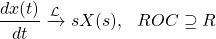where the resulting ROC contains the original ROC.

### Differentation in Frequency-Domain

The derivative in the Frequency-Domain yields the following property: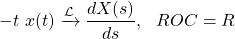which is quite useful in lots of applications.

### Integration in Time-Domain

Integration is more common to be used in the Time-Domain. The Laplace Transform of the integral of signal x(t) is given by: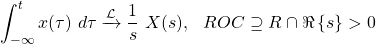where the ROC includes the previous ROC and the positive values of the real part of s.

### Initial and Final Value Theorems

Lastly, there are also two quite important theorems that have to be mentioned.

The initial value theorem is defined as: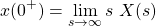where the following conditions have to be met: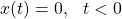and no pulses or high-order anomalies should be in the beginning of x(t).

In addition to this theorem, ifandhas a finite limit for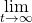, the so called final value theorem defines that: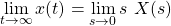## RESOURCES:

### Images

Block diagrams and other visualizations were made using draw.io and GeoGebra

## Final words | Next up

And this is actually it for today's post!

Next time we will get into how LTI systems can be analyzed using the Laplace Transform...

See Ya!Keep on drifting!